# Civil Engineering - GATE Exam Questions

### Exercise :: GATE Exam Questions - Section 4

21.

A point load of 700 kN is applied on the surface of thick layer of clay. Using Boussinesq's elastic analysis, the estimated vertical stress (σv) at a depth of 2 m and a radial distance of 1.0 m from the point of application of the load, is :

 A. 47.5 kPa B. 47.6 kPa C. 47.7 kPa D. 47.8 kPa

Explanation:

No answer description available for this question. Let us discuss.

22.

The liquid limit (LL), plastic limit (PL) and shrinkage limit (SL) of a cohesive soil satisfy the relation

 A. LL > PL < SL B. LL > PL > SL C. LL < PL < SL D. LL < PL > SL

Explanation:

No answer description available for this question. Let us discuss.

23.

A propped cantilever beam of span L, is loaded with uniformly distributed load of intensity w/unit length, all through the span. Bending moment at the fixed end is,

 A.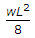B.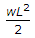C.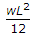D.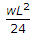Explanation:

No answer description available for this question. Let us discuss.

24.

The sequent depth ratio of a hydraulic jump in a rectangular horizontal channel is 10.30. The Froude Number at the beginning of the jump is :

 A. 5.64 B. 7.63 C. 8.05 D. 13.61

Explanation:

No answer description available for this question. Let us discuss.

25.

Shear centre for an angle-purlin as shown in the below figure is located at,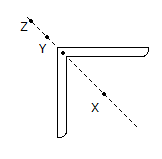A. X B. Y C. Z D. none